# Understanding Earned Value Management: Part 4 Forecasting Equations for a Project

### PMBOK®Guide Definition:

Earned Value Management (EVM): A methodology that combines scope, schedule, and resource measurements to assess project performance and progress.

Estimate at Completion (EAC): The expected total cost of completing all work expressed as the sum of the actual cost to date and the estimate to complete.

Estimate to Complete (ETC): The expected cost to finish all the remaining project work.

Variance at Completion (VAC): A projection of the amount of budget deficit or surplus, expressed as the difference between the budget at completion and the estimate at completion.

To-Complete Performance Index (TCPI): A measure of the cost performance that is required to be achieved with the remaining resources in order to meet a specified management goal, expressed as the ratio of the cost to finish the outstanding work to the remaining budget.

### Practical Definition:

Earned Value Management (EVM): A set of mathematical formulas for determining current project performance and progress and used to forecast anticipated results; strikes the greatest fear in PMP® aspirants seeking certification.

Estimate at Completion (EAC): The new total budget value calculated during project execution.

Estimate to Complete (ETC): The amount of budget needed from the current point in the project to the new estimated completion.

Variance at Completion (VAC): The difference between the original budget amount and the newly calculated budget needed; the difference between BAC and EAC.

To-Complete Performance Index (TCPI): The CPI to be achieved to meet the BAC of the project.

Note: This is the fourth article in a series describing Earned Value Management (EVM). The first article uses a conceptual model describing the current state of a project. The second article uses a conceptual model describing the forecasting of the project. The third article defines the mathematics behind the current project state conceptual model of the first article. This article puts the mathematics behind the second article’s conceptual model for forecasting the project. The fifth article provides the bigger picture of Earned Value Management within the context of the overall project.

### Equations for Forecasting the Project

The current state of our example project is depicted below. We see our progress (EV) is lagging behind our planned value and our actual costs are way over budget. As a result, we predict we’ll spend more (EAC) than our original budget (BAC).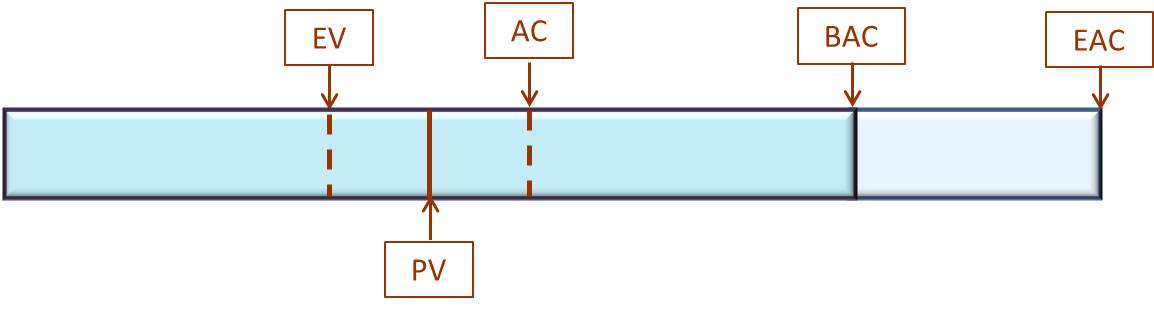Our Sample Project For EVM Discussion

From the third article, let’s provide some numbers for clarity:

BAC= \$10,000
EV  = \$4,000
PV  = \$5,000
AC  = \$6,000

We calculated from those values the following variances and performance indexes:

CV  = -\$2,000
SV  = -\$1,000
CPI = .667
SPI = .8

We can see from the calculated values (and our diagram), costs are more out-of-line than our schedule.

For this project, we need to request more money to complete the scope of the project. How much more money?

The first thing we might do is determine the new total cost of the project, a.k.a., Estimate at Completion (EAC). From the PMBOK® Guide, we see four equations exist for EAC depending on the situation:

### Scenario 1: Progress Will Continue As It Has Been

We use the following equation if progress and spend rate will not change either due to increased resources, scope change, etc. The equation is

EAC = BAC / CPI

Notice how, if CPI is less than 1, EAC will be greater than BAC (simple math – any number divided by a decimal less than one will result in a larger value). If CPI is greater than one, EAC will be less than BAC (remember from the third article, CPI greater than 1 means we are under-budget).

For our example,

EAC = (\$10,000 / .667) or \$15,000 .

Based on this scenario, the total budget for this project is estimated to be \$15,000.

### Scenario 2: Future Work Will Be Completed According to Planned Rate

In this scenario, we change the way we work by working according to the planned rate. If we planned an activity to be 3 days, it will take 3 days, instead of the 4 days under scenario 1’s rate.  We are going back to the plan. Here, we simply take the cost of the remaining work to be accomplished and add it to what we’ve already spent, as illustrated below.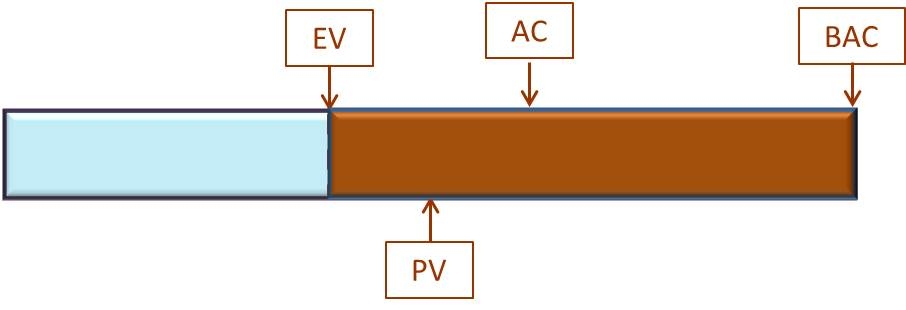Earned Value Management – Calculating EAC used Planned Rate

Breaking it down, BAC – EV represents the cost of the remaining work:

Remaining work = \$10,000 – \$4,000 or \$6, 000. Add that to what we’ve spent so far (AC) and we have our new EAC:

EAC = AC + remaining work or \$6,000 + \$6,000 = \$12,000. Using substitution, the equation is

EAC = AC + BAC – EV

EAC = \$6,000 + \$10,000 – \$4,000 = \$12,000.

### Scenario 3: The Initial Plan No Longer Valid

In this scenario, something has caused the initial plan to become invalid. It could be a power outage, hurricane, federal regulations changes or new laws, workers strike, death of a key person, etc. Something has had a major impact on the plan. Or, we simply say the original plan is not workable and decide to scrap and re-plan based on our new knowledge and the remaining work to be done. In order to recover, the key stakeholders request a new plan and new estimate at completion. They still want the original scope met along with the additional scope added (clean-up from the storm, finding a replacement for the key person, etc.). Here is the equation:

EAC = AC + Bottom-up ETC

Here’s an illustration: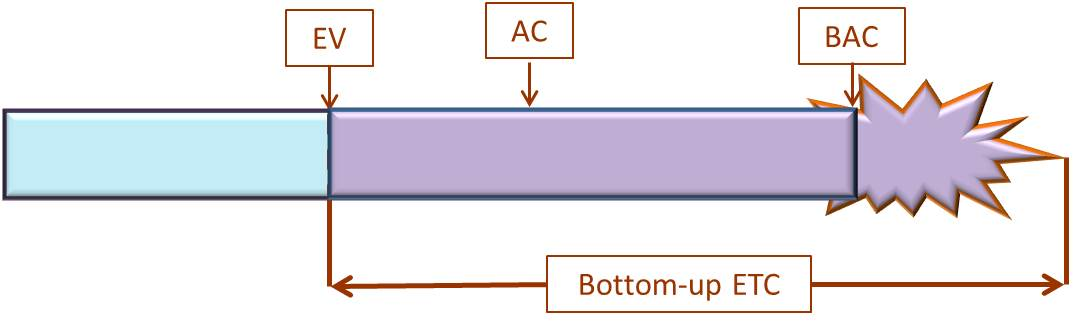Earned Value Management – Calculating EAC using Bottom-up ETC

Of course, we have already spent the original AC, so we need to add that into the total of the project cost. We re-plan our work, determine a new schedule and a new budget. Let’s say the new budget equals \$10,000 based on the new plan. We determine that new budget using bottom-up estimating, thus the term Bottom-up Estimate To Complete or Bottom-up ETC.

Bottom-up ETC = \$10,000.

EAC = \$6,000 (AC) + \$10,000 (Bottom-up ETC) = \$16,000.

### Scenario 4: Schedule and Cost Performance Impact the Remaining Work

In this case, both the schedule performance and the cost performance impact the plan. Two factors have occurred during our project execution: new technology or an alternate method is discovered which drastically reduces the time required to complete the project or a request to add to the scope of work, and the cost of materials not yet acquire has suddenly tripled in price. In these cases, both the SPI and CPI would influence the EAC.

EAC = AC + [(BAC – EV) / (CPI x SPI)]

• AC is what we’ve spent so far (also known as sunk costs).
• (BAC – EV) is the remaining work based on current estimates.
• (CPI x SPI) are the factors which impacts the remaining work.

Using our current values,

EAC = \$6,000 + [(\$10,000 – \$4,000) / (.667 * .8)] or \$6,000 + (\$6,000/.534) = \$6,000 + \$11,236 = \$17,236.

### Which EAC?

As we see, each method of calculating EAC produces a different value.

• Scenario 1: \$15,000
• Scenario 2: \$12,000
• Scenario 3: \$16,000
• Scenario 4: \$17,236

Before jumping to the conclusion of going with the highest value and then returning the extra money if not used, consider your reputation and credibility. In many companies, requesting more money and returning it later is frowned upon. The “extra” money could have been put to use elsewhere and your reputation can be damaged. Estimate according to the situation as described above and you’ll be better off.

For PMP® aspirants, read the exam question carefully. It is state the situation and ask you to calculate the new EAC using the right formula. If you don’t use the proper formula, your answer will not match any selection provided. Remember, each question on the exam is worth only 1 point. Do your best, but don’t waste too much time doing and re-doing your answer. It is better to guess at a number and move on rather than lose too much time and not completing the exam. We’ve warned many students about fretting over one problem and running out of time. Just Don’t Do It.

### What’s Estimate to Complete (ETC)

Now that we understand EAC and estimated the total project cost at completion, we need to determine how much money we need to complete the project from this point. That value is Estimate to Complete (ETC).

ETC = EAC – AC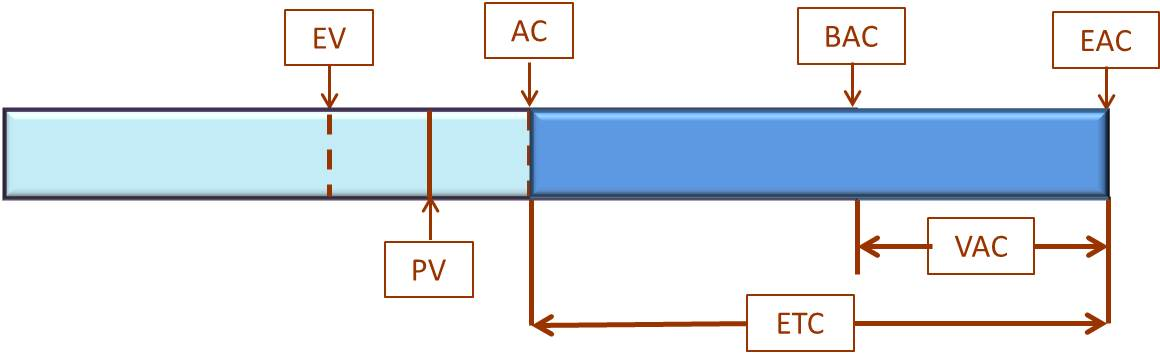Earned Value Management – Estimate to Complete Illustration

Estimate to Complete is simply the new Estimate at Completion (EAC) minus what we’ve already spent (AC). Let’s assume we have are Scenario 1 from above and EAC = \$15,000.

ETC = \$15,000 – \$6,000 or \$9,000

From this point in the project, we will need a total of \$9,000 to complete the remaining work. Of course, if we are in scenario 3, we already know ETC. We determined it by calculating Bottom-up ETC.

### Variance at Completion (VAC)

Variance at Completion is a fancy term for “What is the difference between the initial budget and our new estimated budget”.

VAC = BAC – EAC

If VAC is negative, we were over-budget. If VAC is positive, we were under-budget. Using our example:

VAC = \$10,000 – \$15,000 or -\$5,000.

We are over-budget by \$5,000.

### To-Complete Performance Index (TCPI)

After all our estimations and calculations, our key stakeholders turn down our request for more time or money. They say we must hold to the budget and timeframe. We need to determine how efficient we must be to meet those constraints. To-Complete Performance Index tells us what we need to do.

TCPI = (BAC – EV) / (BAC – AC)

Simply put, the equation is the remaining work divided by the remaining budget.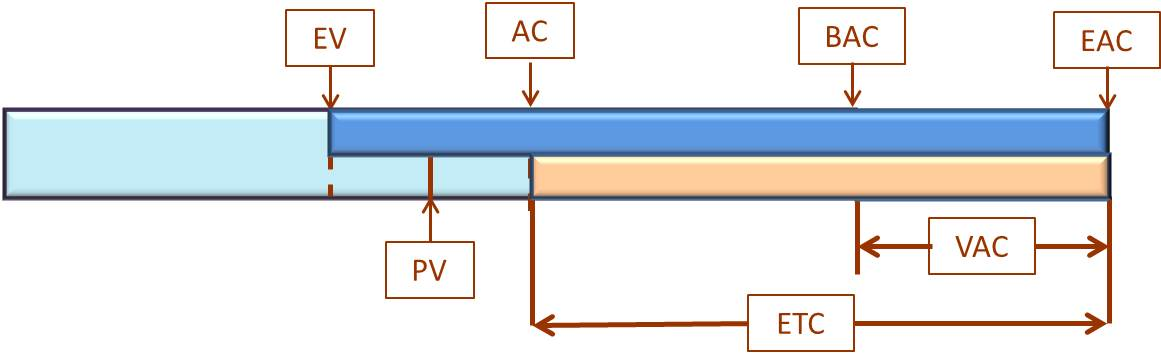Earned Value Management – To-Complete Performance Index Illustration

Although the equation makes it look complex, we do this calculation in our heads for home projects. We look at the remaining work and what’s left in the budget and say, “ok, we need to complete this project with this amount of money.” So, we boost our work performance, hire cheaper labor, look for items on sales, etc. Using our example:

TCPI = (\$10,000 – \$4,000) / (\$10,000 – \$6,000) which equals \$6,000 / \$4,000 or 1.5.

We must increase our current CPI from .667 to 1.5 in order to complete this project on-time and on-budget. That is a huge improvement and we doubt, feasible. The greater TCPI is above 1, the harder it will be to complete within the constraints. The smaller the value, the easier it is.

If we are granted additional time and money, we use a different equation. Let’s choose EAC from scenario 2: \$12,000.

TCPI = (BAC – EV) / (EAC – AC)

TCPI = (\$10,000 – \$4,000) / (\$12,000 – \$6,000) or \$6,000 / \$6,000 = 1.

In this case, we need to perform at our planned rate and we will finish within the new EAC value.

### One Final Note

Earned Value Management and its calculations describe the state of a project at a particular point in the project execution. As progress continues, the values in EVM should be recalculated. The values from a month ago are no longer valid; time does not stand still. In the event we’ve calculated a new EAC and are granted additional funds, the EAC becomes the BAC for the next round of calculations. The original budget no longer exists because it has been replaced by the new, approved budget value. All future EVM calculations will reflect these budget values.

### Conclusion

In this article, we developed the mathematics behind the forecasting a project’s future. The diagrams attempt to illustrate the formulas. We used a variety of examples and scenarios to best cover the formula.

In the next article, we further understand Earned Value Management and how it relates to the Work Breakdown Structure. With the understanding, we can use EVM in our real-world project environments.